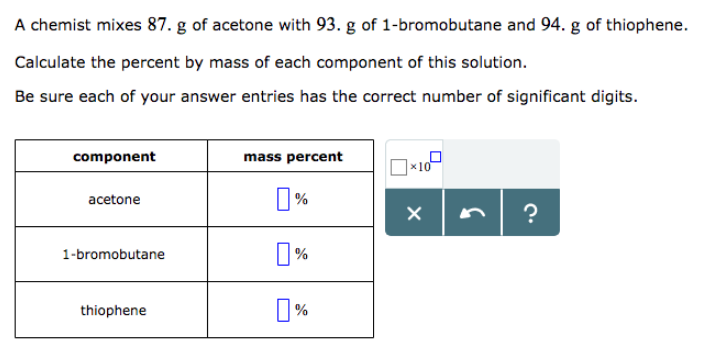# Problem: A chemist mixes 87. g of acetone with 93. g of 1-bromobutane and 94. g of thiophene. Calculate the percent by mass of each component of this solution. Be sure each of your answer entries has the correct number of significant digits.

###### FREE Expert Solution
91% (330 ratings)###### Problem Details

A chemist mixes 87. g of acetone with 93. g of 1-bromobutane and 94. g of thiophene.

Calculate the percent by mass of each component of this solution.

Be sure each of your answer entries has the correct number of significant digits.Frequently Asked Questions

What scientific concept do you need to know in order to solve this problem?

Our tutors have indicated that to solve this problem you will need to apply the Mass Percent concept. You can view video lessons to learn Mass Percent. Or if you need more Mass Percent practice, you can also practice Mass Percent practice problems.

What professor is this problem relevant for?

Based on our data, we think this problem is relevant for Professor Abeykoon's class at UTD.